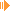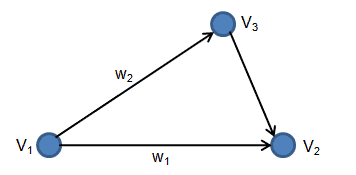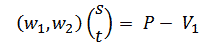Point in Triangle AlgorithmsDownload Article and Source (39 kb)

I. Introduction

This article is about how to check if a point is inside a given triangle or not. Two approaches are shown and also implemented exemplary as Java applet. This description targets the twodimensional case.

So given is a triangle T with its three vertices V1= (vx1, vy1), V2= (vx2, vy2), V3= (vx3, vy3) and a testpoint P = (px, py).
Question: Is P included in T?

II. Algorithm 1: "Crossproduct Side Algorithm"

Note that above name of this algorithm is not official, but my own invention (hope you like this name!). Note that following topic is quite similar to the discussion in my article about polygon convexity , but here we have a look from the other side.

Maybe you ask yourself now how the crossproduct is defined in 2D and how to interpret it geometrically? Well, the crossproduct is actually not well defined for the 2D case, but a common definition is

 perpDotProduct(v1, v2) = vx1 * vy2 - vy1 * vx2

This is also known as 'perp dot' product and has some nice properties - if you are interested, read . Also note that it returns a scalar and not a vector (in contrast to the 3D crossproduct) and is actually the same as the determinant of the two two-element vectors. From the latter fact, one (with that I mean a good mathematician) can derive following useful properties:

 perpDotProduct(v1, v2) = 0   => v1, v2 are parallel perpDotProduct(v1, v2) > 0   => v2 is clockwise from v1 perpDotProduct(v1, v2) < 0   => v1 is counterclockwise from v2The figure on the left shows the perpDotProduct result geometrically.

So actually this all we need :) Calculate the perpDotProduct/crossproduct of all three points V1, V2, V3 with the testpoint P. (It's important that P is either always the first argument or either always the second argument, otherwise you might get into trouble in the final check below!)
Call c1 = crossproduct(V1, P), c2 = crossproduct(V2, P) and c3 = crossproduct(V3, P).
The final point-in-triangle depends on the prior knowledge of the triangle:

• if you know that the vertices V1, V2, V3 are given in clockwise order, then P is inside the triangle if
c1> 0 && c2> 0 && c3 > 0.
• if you know that the vertices V1, V2, V3 are given in counterclockwise order, then P is inside the triangle if
c1< 0 && c2< 0 && c3 < 0.
• if you do know nothing about the given order of vertices V1, V2, V3, then P is inside the triangle if
(c1> 0 && c2> 0 && c3 > 0) || (c1< 0 && c2< 0 && c3 < 0).

III. Algorithm 2: "Barycentric Algorithm"

The barycentric approach is based on another mathematical point of view. Imagine that the triangle is part of a plane -that means all three vertices of the triangle lie inside this plane. So we can pick one vertice and the two edges of the triangle starting from this vertices span the whole plan:Define w1 = V2 - V1 and w2 = V3 - V1. Then each point in the plane can be written as: P(s,t) = V1 + s * w1 + t * w2      s,t e R By limiting s and t by s >= 0, t >= 0 and (s + t) <= 1, then P(s,t) describes exactly the points inside the triangle!

So have an equation that gives us an arbitrary point inside the traingle by choosing values for s and that fulfill the three limitations s >=0, t >= 0 and (s + t) <= 1.
This means that for our testpoint P, we can describe it with this equation P(s,t) - and if s and t fulfill the requirements than P is inside T. Obviously, our goal is to calculate s and t for testpoint P -

 V1 + s * w1 + t * w2 = P

Rearranging gives

 s * w1 + t * w2 = P - V1

or in vector notation:Either we solve this by hand or apply Cramer's Rule  (note that for our special 2D case the determinant is calculated equally as the perpDotProduct!):

 s = determinant(P - V1, V2) / determinant(V1, V2) t = determinant(V1, P - V2) / determinant(V1, V2)

The final point-in-triangle is then

• P is inside the triangle if s >= 0 && t >= 0 && (s + t) <= 1.

That's it. Hope you enjoyed this article and find the applet + source useful!

References:

Sunshine, December 2011

This site is part of Sunshine's Homepage